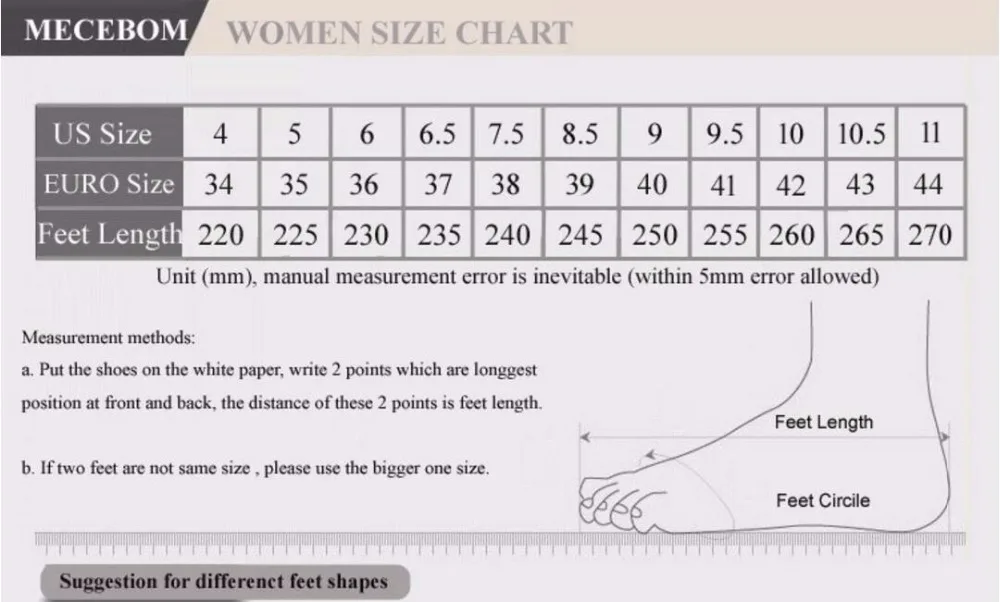DELOCRD

## DELOCRD Faux Fur Short Boots

Regular price \$50 now \$29 Unit price  per

Shipping calculated at checkout.

• Faux Suede
• Faux Fur
• Ankle Height
• Slip On Design
• Shock Absorbing
• Square Heel
• Round Toe
• Rubber Out-Sole
• Snow Boots

Size Guide34 = 22cm = 4 United States
35 = 22.5cm = 5 United States
36 = 23cm = 6 United States
37 = 23.5cm = 6.5 United States
38 = 24cm = 7.5 United States
39 = 24.5cm = 8.5 United States
40 = 25cm = 9 United States
41 = 25.5cm = 9.5 United States
42 = 26cm = 10 United States
43 = 26.5cm = 10.5 United States
44 = 27cm = 11 United States
45 = 27.5cm = 11.5 United States
46 = 28cm = 12 United States
47 = 28.5cm = 12.5 U.S.
48 = 29cm = 13 United States